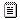## NXT PROBLEM

Discussion specific to projects ideas and support.
naneczek
Posts: 28
Joined: 08 Jun 2013, 10:09

### NXT PROBLEM

I have attached bricx's algorithms and I stopped at a certain point. How can I modify/add to this code sth that will display on a screen the process of the distance frequency changing with one hundred measurements per second frequency. Each measurement is represented by a point on a screen and each measurement is supposed to be saved in an array and after that it would go back to the measurement mode. I hope this description is clear. There should be a loop and so on. Can anyone do this?
AttachmentsUntitled66.nxc
nxc
(169 Bytes) Downloaded 352 times

HaWe
Posts: 2500
Joined: 04 Nov 2014, 19:00

### Re: NXT PROBLEM

you can post your code using the code tags!

Code: Select all

``````task main() {
ClearScreen();
int x;
int i;

SetSensorLowspeed(IN_4);
x = SensorUS(IN_4);
for (i=0;i<100;i++) {

PointOut(i,i);
}
while (true){
}
}

``````

HaWe
Posts: 2500
Joined: 04 Nov 2014, 19:00

### Re: NXT PROBLEM

the last while() needs a ; but don't need an extra { }.

In the loop you will have to poll the sensor each time.

Sensor US gives you just 1 reading about every 20ms because it's an i2c sensor.

The NXT screen x axis is 100 pixels wide, the y axis is about 70 pixels high.

assuming your US reading is up to 255 then you'd take 1/4 of your USS readings to fit to your y axis.

sth like this should do what you expected:

Code: Select all

``````task main() {
ClearScreen();
int y;
int i;

SetSensorLowspeed(IN_4);

for (i=0;i<100;++i) {
y = SensorUS(IN_4);
PointOut(i, y/4, DRAW_OPT_NORMAL);
NumOut(0,56, y, DRAW_OPT_CLEAR_EOL);
Wait(1);
}

while (true);
}``````

HaWe
Posts: 2500
Joined: 04 Nov 2014, 19:00

### Re: NXT PROBLEM

ps
as the TOP is more a software issue it's supposed to be better located to the "Mindstorms Software" subforum!

naneczek
Posts: 28
Joined: 08 Jun 2013, 10:09

### Re: NXT PROBLEM

Thank You. Will this algorithm work like an oscyloscope? Measuring the distance from the object?

HaWe
Posts: 2500
Joined: 04 Nov 2014, 19:00

### Re: NXT PROBLEM

you will have to reset i to 0 within the loop if it exceeds 100, and then to clear the screen,
but then: yes, I think so.

just add the line
if (i>=100) {i=0; ClearScreen();}
edit
as the 1st one in your loop.

And for you as a beginner just 1 extra hint:
for the start, ever use ++i or i+=1 instead of i++ unless you once will know exactly the difference.
(i++ may have unexpected side effects in several programs if you don't know how to handle this.)

HTH!
Last edited by HaWe on 08 Jun 2013, 12:00, edited 1 time in total.

naneczek
Posts: 28
Joined: 08 Jun 2013, 10:09

### Re: NXT PROBLEM

task main() {
ClearScreen();
int y;
int i;

SetSensorLowspeed(IN_4);

for (i=0;i<100;++i) {
y = SensorUS(IN_4);
PointOut(i, y/4, DRAW_OPT_NORMAL);
NumOut(0,56, y, DRAW_OPT_CLEAR_EOL);
Wait(1);
if (i>=100) {i=0; ClearScreen();}
}

while (true);

Sth like this? But shouldn't I use the clausule "loop" there anywhere?

HaWe
Posts: 2500
Joined: 04 Nov 2014, 19:00

### Re: NXT PROBLEM

please use code tags!!

srry, I actually meant: as the 1st line in your loop, and you additionally will have to modify the loop condition:

so sth like this:

Code: Select all

``````task main() {
ClearScreen();
int y;
int i;

SetSensorLowspeed(IN_4);

for (i=0;i<=100;++i) {
if (i>=100) {i=0; ClearScreen();}
y = SensorUS(IN_4);
PointOut(i, y/4, DRAW_OPT_NORMAL);
NumOut(0,56, y, DRAW_OPT_CLEAR_EOL);
}

while (true);
}
``````

naneczek
Posts: 28
Joined: 08 Jun 2013, 10:09

### Re: NXT PROBLEM

I've got another question. How to implement an array in this code which will save 100 measurements on the display, wait one second and repeat the measurements??

Sth like this isn't working:
task main() {
ClearScreen();
int y;
int i;
int MyArray[];

SetSensorLowspeed(IN_4);
ArrayInit(MyArray,0,100);
for (i=0;i<=100;++i) {
if (i>=100) {i=0; ClearScreen();}
y = SensorUS(IN_4);
PointOut(i, y/4, DRAW_OPT_NORMAL);
NumOut(0,56, y, DRAW_OPT_CLEAR);
}

while (true);
}

HaWe
Posts: 2500
Joined: 04 Nov 2014, 19:00

### Re: NXT PROBLEM

hey,

please use code tags for your code!

simple and stupid:

Code: Select all

``````// *SNIP*

int myUSSvalues;   // <<<<<<<<<<<<<<<<<<<<<<<<<<<<<

for (i=0;i<=100;++i) {
if (i>=100) {i=0; ClearScreen();}
y = SensorUS(IN_4);

myUSSvalues[i]=y;   // <<<<<<<<<<<<<<<<<<<<<<<<<<<<<

PointOut(i, y/4, DRAW_OPT_NORMAL);
NumOut(0,56, y, DRAW_OPT_CLEAR_EOL);

if (i==99) Wait(1000);    // <<<<<<<<<<<<<<<<<<<<<<<<<<<<<
}

// *SNIP*
``````
;)
Last edited by HaWe on 15 Jun 2013, 16:00, edited 1 time in total.

### Who is online

Users browsing this forum: No registered users and 4 guests